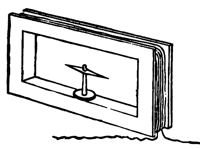17,275 PagesCurrent can be measured by a galvanometer, via the deflection of a magnetic needle in the magnetic field created by the current.

The ampere (symbol: A) is the SI unit of electric current (symbol: I) and is one of the seven SI base units. It is named after André-Marie Ampère (1775–1836), French mathematician and physicist, considered the father of electrodynamics. In practice, its name is often shortened to amp.

In practical terms, the ampere is a measure of the amount of electric charge passing a point in an electric circuit per unit time with 6.241 × 1018 electrons, or one coulomb per second constituting one ampere.

The practical definition may lead to confusion with the definition of a coulomb (i.e., 1 amp-second), but in practical terms this means that measures of a constant current (e.g., the nominal flow of charge per second through a simple circuit) will be defined in amps (e.g., "a 20 mA circuit") and the flow of charge through a circuit over a period of time will be defined in coulombs (e.g., "a variable-current circuit that flows a total of 10 coulombs over 5 seconds"). In this way, amperes can be viewed as a "rate of flow" and coulombs viewed as an "amount of flow."

## References / sources

1. BIPM official definition
2. The other six are the metre, kelvin, second, mole, candela, and kilogram
3. Bodanis, David. (2005). Electric Universe. New York: Three Rivers Press. ISBN 978-0307335982

Extract from Wikipedia article.

Community content is available under CC-BY-SA unless otherwise noted.